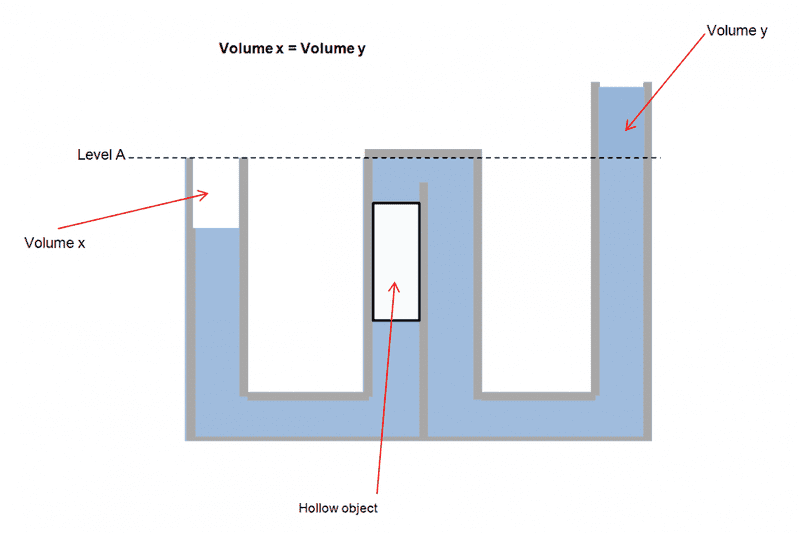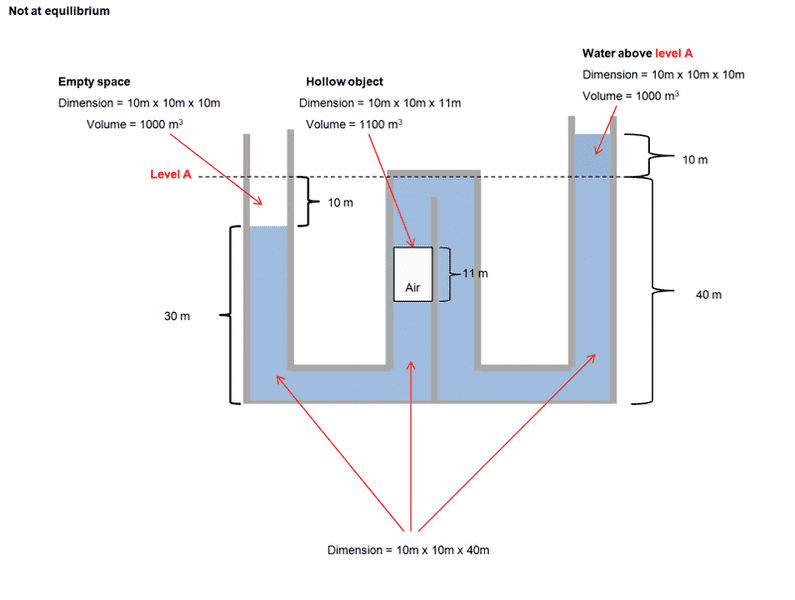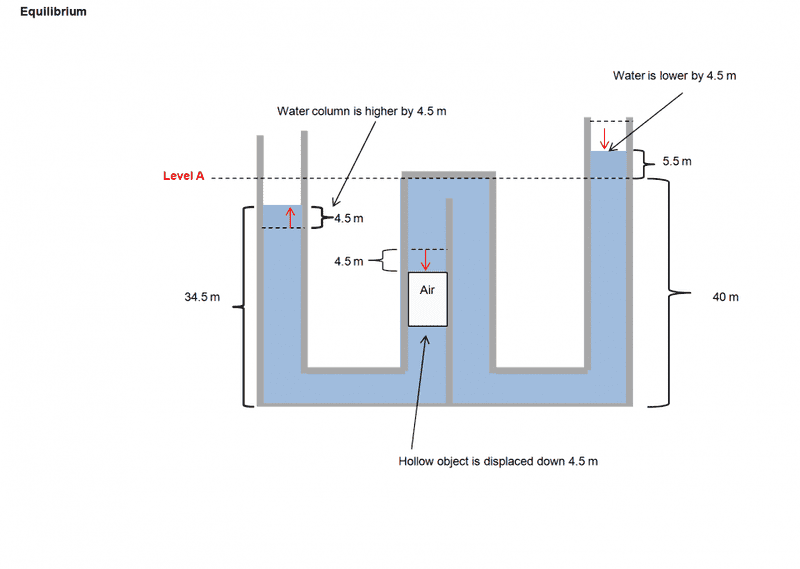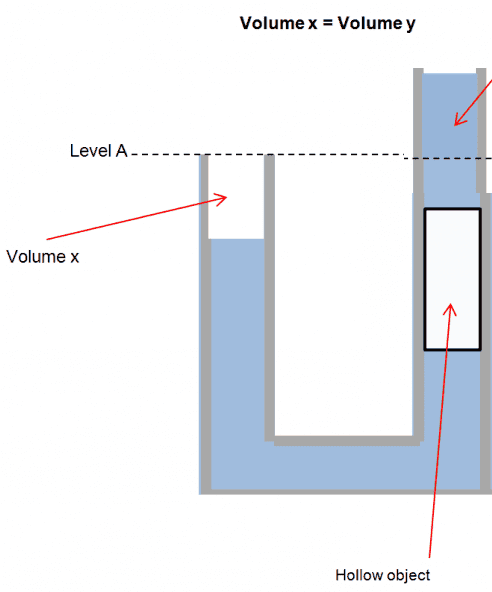# Water column pressure question

In the diagram I included, I was curious as to a this situation.

The columns of water are all connected and there is a hollow object that is buoyant and it's walls hug up against the walls of the left side of the 2 middle columns so that no water cannot pass between the two walls --- the hollow object can slide up and down the column.

My question is - since the far right column has a higher height of water (thus higher pressure), in a normal situation (without the hollow object present) the water on the right will push through to the left column and the water in the far left column would rise to the level "A". But with the hollow object placed where it is -- there is no difference? Even though the object is hollow, it will be pushed down by the greater water pressure on the far right column until the water in the left column rises to level "A"?#### Attachments

andrewkirk
Homework Helper
Gold Member
Your last sentence is correct. The difference between the water pressure on the top of the object and that on the bottom will move it until the two ends are at equal levels.

In fact, it will overshoot so that the left end water level will go a little higher than the right. It will then come back, undergoing damped oscillation around the equilibrium point, but friction will ensure it soon settles at the equilibrium point.

sophiecentaur
Gold Member
Your last sentence is correct. The difference between the water pressure on the top of the object and that on the bottom will move it until the two ends are at equal levels.

In fact, it will overshoot so that the left end water level will go a little higher than the right. It will then come back, undergoing damped oscillation around the equilibrium point, but friction will ensure it soon settles at the equilibrium point.

I don't think that's right for all cases and there are no numbers quoted in the problem.
The presence of the buoyant 'piston' must have an effect. Equilibrium will be reached when the three forces on the piston (two pressures and its weight) sum to zero. The left hand U situation and the right hand U situation are both affected by the presence of the piston. The solution to this problem can only be 'proved' by actually working it out, I think.
For a massless 'bubble' in place of the piston, equilibrium would be reached when the difference between the heights in the left hand section is equal to the difference in heights in the right hand section. Forces on the top and bottom of the bubble will be equal and opposite. That will depend on the detailed column and bubble lengths.

On the subject of the damped oscillation, the details will depend on the viscosity, friction, liquid density and tube diameter. The damping will be strong, in any practical situation.

•gloo
andrewkirk
Homework Helper
Gold Member
The presence of the buoyant 'piston' must have an effect.
How embarrassing, you are so right! I had not thought about the weight of the piston.

I think that if the piston, including its interior, has the same average density as the fluid then the equilibrium will have both ends at the same level. If it is denser (less dense) than the fluid, equilibrium will have the left side being higher (lower) than the right.

•gloo
sophiecentaur
Gold Member
How embarrassing,
Not at all. We all appreciate these sorts of questions because they make us think and shooting from the hip is quite fair enough - it gets things going.

Yes - if the bubble is of the same substance, what you say must be right; both ends will be at the same level.

•gloo
Not at all. We all appreciate these sorts of questions because they make us think and shooting from the hip is quite fair enough - it gets things going.

Yes - if the bubble is of the same substance, what you say must be right; both ends will be at the same level.

I showed the object being hollow and buoyant...but where you referring to that thing being the same density as the water?

sophiecentaur
Gold Member
I showed the object being hollow and buoyant...but where you referring to that thing being the same density as the water?
There are three possible cases for the object. More Dense, Less dense and the same density as the water. Your original scenario was just one of them. I was just pointing out that, only for a specific gravity of 1 would the levels become the same.

•gloo
andrewkirk
Homework Helper
Gold Member
A concise way to look at it is by considering the forces on the bottom face of the piston object. The upward force on that face is equal to
$$A\rho h_1g$$
where ##A,\rho,g## and ##h_1## are respectively the area of the face, the density of the fluid, the accel due to gravity and the height of the left-side water level above the face. The downward force on that face is equal to the weight of the object plus the downward force on the top of the object, which is equal to
$$A\rho' hg+A\rho h_2g$$
where ##\rho', h## and ##h_2## are respectively the average density of the object, the height of the object and the height of the right-side water level above the top face of the object.

At equilibrium, these two forces must be equal in magnitude, which gives

$$\rho(h_1-h_2)=\rho' h$$
The excess of the left-side water level over the right will be

$$h_1-(h_2+h)=h\left(\frac{\rho'}\rho-1\right)$$
We see from this formula that the heights will be equal if the object is denser than the fluid and the left side will be higher (lower) if the object is denser (less dense) than the fluid.

•gloo
Keith_McClary
Gold Member
Can it be simplified like this?:
https://www.physicsforums.com/attachments/utube-png.101828/?temp_hash=9ecf222be4cb0ec656ef94632f5e6ef9 [Broken]

Last edited by a moderator:
A concise way to look at it is by considering the forces on the bottom face of the piston object. The upward force on that face is equal to
$$A\rho h_1g$$
where ##A,\rho,g## and ##h_1## are respectively the area of the face, the density of the fluid, the accel due to gravity and the height of the left-side water level above the face. The downward force on that face is equal to the weight of the object plus the downward force on the top of the object, which is equal to
$$A\rho' hg+A\rho h_2g$$
where ##\rho', h## and ##h_2## are respectively the average density of the object, the height of the object and the height of the right-side water level above the top face of the object.

At equilibrium, these two forces must be equal in magnitude, which gives

$$\rho(h_1-h_2)=\rho' h$$
The excess of the left-side water level over the right will be

$$h_1-(h_2+h)=h\left(\frac{\rho'}\rho-1\right)$$
We see from this formula that the heights will be equal if the object is denser than the fluid and the left side will be higher (lower) if the object is denser (less dense) than the fluid.
Thank you for the input. I am going to put up the diagram and put some numbers and see if my logic makes sense very shortly

So for the query I have asked I redrew the diagram and tried to put up some numbers to see if my logic makes sense.

For the solving of the problem I made some assumptions:

1. The blue liquid is water with a density of 1000 kg/ cubic meter
2. The gravity constant used will be 10m/ second squared (rounded for simplicity)
3. The hollow object in the middle column is rigid and does not compress and is full of air
4. The material making up the empty object is assumed mass less (for simplicity)
5. The hollow object can slide with zero friction up or down the water column and not allow water to pass between it's walls and the wall of the column
6. Each column has an area of 10m by 10m (looking down on the columns)
7. The heights of the water in the column is shown in diagram 1.

Thought process: (this is not exactly scientific but just how I did the logic in my head)

A. There is an imbalance in pressure in the columns and the far right column has greater pressure at the bottom than the other columns to the left
B. The middle left column where the hollow object is will only be able to have a constant height of water equal to 29 m.
C. The water in the far right column will move down because of the greater pressure (due to the extra 10 meters of height) -- the pressure transfers to the left columns undiminished until equilibrium is reached
D. the hollow object gets pushed down by the excess pressure coming from the far right column
E. Equilibrium is reached when 4.5m of height in the far right column gets transferred due to greater pressure -- to the far left column
F. The final height of water in each column is as follows:

Far left column -- 34.5 m (just below Level A)
Middle left column 29 m (same as before but hollow object is pushed lower)
Far right column -- 45.5 m (4.5 meters transferred to left columns)

My logic concludes that at these heights of water, the 5.5 meter of water above level A adds just enough pressure to the column with the hollow object to push the water up in the far left column to 34.5 meters in height. The hollow object is pushed down lower of course.

Pressure in far left column = height * gravity * density
= 34.5 *10 m/second square * 1000 Kg/cubic meter
= 345,000 Pa

Pressure in middle left column = height of water in column + (added pressure from far right column)
= (29 * 10 * 1000) + (5.5 *10 * 1000)
= 345,000 Pa

Thus, when 4.5 meters of water is transferred from the far right column (due to greater pressure) to the far left column, it will reach equilibrium.

I know I am sloppy with the explanation and the in showing the math...but is this more or less correct in terms of the final equilibrium?

The diagrams are below to kind of illustrate my question

Diagram 1 (non equilibrium)Diagram 2 ( at equilibrium)andrewkirk
Homework Helper
Gold Member
That result looks correct to me.

I like your diagrams. What did you use to create them?

•gloo
That result looks correct to me.

I like your diagrams. What did you use to create them?

Thanks for all your time and effort in helping me Andrew. The diagrams are just done with plain old Microsoft Power Point.

sophiecentaur
Gold Member
The diagrams are just done with plain old Microsoft Power Point.
..and they are just what's needed for almost all simple pictures. I am always amazed that so many contributors don't bother to use it (or one of the many lookalikes). That awful 'Paint' is about all that many people manage to use. And many of them are very familiar with computers and apps.
Keep it up and everyone will appreciate it.

•gloo
Keith_McClary
Gold Member
Can it be simplified like this?:
https://www.physicsforums.com/attachments/utube-png.101828/?temp_hash=9ecf222be4cb0ec656ef94632f5e6ef9 [Broken]

Last edited by a moderator:
Keith_McClary
Gold Member
Does the same analysis apply to a U-tube?(My image didn't display in previous post).

andrewkirk
Homework Helper
Gold Member
Does the same analysis apply to a U-tube?
Yes the analysis is the same, as is the result. The shape and maximum level of the water between the two ends does not affect the calculation, only the relative levels of the two ends.

That's why siphoning works - a phenomenon I rely on to get my washing machine water out onto the garden when we are having a drought, without having to carry it bucket by bucket.

Keith_McClary
Gold Member
Assuming unit cross-sectional area (of the column) and water density, the Potential Energy of the water above the line is ##h^2/2##. The PE of the missing water below the line on the left is the same. The PE of the missing water in the bubble is ##L(d - h)##, where ##L## = length of bubble and ##d## is its depth. So we want to minimize ##h^2 + L (d - h)##, which gives ##h=L/2##.

..and they are just what's needed for almost all simple pictures. I am always amazed that so many contributors don't bother to use it (or one of the many lookalikes). That awful 'Paint' is about all that many people manage to use. And many of them are very familiar with computers and apps.
Keep it up and everyone will appreciate it.
Thank you so much for your help and contribution as well :)

Gloo touched on friction of the "bubble" moving in assumption 5. Friction has to be considered for it. That friction will keep the bubble from allowing the water to equalize in both columns. Also, the density of the bubble will make a difference though gloo stated it boyuant. If it is lighter than water as stated it will make no difference. But, it could be heavy enough to counter balance the water in volume Y pulling it below level A.

Gloo touched on friction of the "bubble" moving in assumption 5. Friction has to be considered for it. That friction will keep the bubble from allowing the water to equalize in both columns. Also, the density of the bubble will make a difference though gloo stated it boyuant. If it is lighter than water as stated it will make no difference. But, it could be heavy enough to counter balance the water in volume Y pulling it below level A.

Yeah..i know it was unrealistic to say no friction...I just assumed it away for simplicity...perhaps if the pressure difference is big enough, the friction as a relation to total force is minimal. For instance, if we know that area is A=pi *r *r, we know that the size of the area increases as a square with increase in diameter. But the circumference is only c=pi *r *2. Thus the friction on the sides only increase as a linear factor?

Water pressure is very strong. Thus, friction to stop it from bypassing bubble has to be strong also. I published my response without showing concern for a "lighter than water" bubble as if it would not make much difference to see what kind of response that would get. But, if a bubble with a lot higher specific gravity was used it could counter balance as I wrote. Therefore, a large bubble, as drawn, with a much lighter specific gravity that the fluid water (or some much heavier specific gravity fluid) would also have to make considerable difference also because it displaces the fluid from the tube it is in.